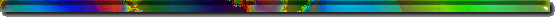Opening Page Science1- Astr/Cosml - Science2- Earth - Science3- Life - Music Visual Arts Humor Law/Politics War G.Warming Nuclear Links DisclaimerRINGNEBULA.COM

CMB NOTES: "3000K Blackbody Calculations"

Gary D. Evans

 Last Updated: April 10, 2019 7:11 AM

_______________________________________________________________________

 About 380,000 years after the "Big-Bang," the Universe cooled to below 3000K at which time protons and electrons were able to combine - forming neutral hydrogen atoms. With the electron density thereby falling significantly, most of the photons no longer scattered repeatedly and were instead free to travel linearly to our detectors -- 13.8 billion years later. What follows is a straight-forward algebraic description of that event. ________________________________________________________________________ EXPERIMENTALLY DERIVED DATA Atomic hydrogen is ionized by 13.6eV photons CMB blackbody microwave radiation peak wavelength = 1.065mm CMB blackbody radiation peak = 2.7255 K ________________________________________________________________________ 1) 13.6eV photons (able to ionize hydrogen) = wavelength 91nm PROOF: 13.6eV photons = wavelength 91nm E=hf (Where h = Planck Constant=6.63 x10-34 Joule*Secs) and c=3x108m/sec E=hc/lambda (Where lambda=wavelength, here = 91x10-9m) E=[(6.63×10-34Joules*sec) × (3×108m/sec)]/(91x10-9 meters) = 2.185714x10-18Joules 1 Joule = 1 Coulomb of charge moved through a potential difference of 1 Volt 1eV is the work of moving 1 electron (charge of 1.60 x 10 -19 Coulombs) through a potential difference of 1 volt. Therefore, an electron moving through 1 volt carries 1.60 x 10-19 Coulombs/volt = 1.60 x 10-19 Joules of energy. As a 91nm photon carries 2.185714x10-18 Joules of energy, and an electron moving through 1 volt carries 1eV of energy [1.60 x 10-19 Joules], a 91nm photon carrying 2.185714x10-18Joules / 1.60 x 10-19 Joules/eV = ~13.6eV of energy. ________________________________________________________________________ 2) Peak 972nm CMB blackbody photons are now received at ~1mm wavelength. PROOF: Current 1.065mm wavelength CMB photons were emitted at ~975nm wavelength The peak CMB LSS photons now received have wavelengths of 1.065mm after being redshifted by z = 1090 from the LSS to the present (i.e., space has expanded by 1090 times since the CMB LSS era. The currently detected 1.065mm photons were 1090 times shorter in wavelength when emitted at the CMB LSS era. 1.065x10-3m / 1.090x103 = 0.9752x10-6m = 975.2nm Recall that hydrogen is ionized by 13.6eV photons at wavelength = 91nm. This wavelength is 10-times too long (and 10-times too low in energy) to ionize hydrogen. However: The CMB LSS blackbody peak wavelength received is a component of a blackbody spectrum as defined by Wien's displacement Law. A blackbody spectrum with peak wavelength of 975nm will contain a sufficient number of higher-energy (shorter wavelength) photons - tailing off at ~90nm. Those higher-energy photons were present in sufficient numbers to provide the needed hydrogen ionization until the system cooled further, at which time the ionized hydrogen (protons) combined with electrons to form atomic hydrogen (neutral). As charged particle density fell rapidly, Thompson scattering of photons largely ended, allowing those photons to travel away from the CMB LSS - reaching us today, essentially freezing an image of that surface. A blackbody spectrum with a peak wavelength of 975nm defines the temperature of the blackbody of ~3000K ________________________________________________________________________ 3) The detected CMB microwave signal of 2.7255K was the result of a shift from ionized hydrogen to neutral hydrogen as the Universe cooled to below 3000K. Space has expanded since that transition, resulting in that LSS blackbody spectrum shifting from 3000K to the currently detected 2.7255K spectrum. ________________________________________________________________________ Summary of above dataand PROOF: Space itself, having expanded over 1000x since the 3000K LSS era, has converted that 3000K-peak blackbody spectrum to one peaking at 2.7225K. Wien's displacement Law: The spectral radiance of a blackbodys radiation per unit wavelength, peaks at the wavelength lambda-max given by lambda-max = b/T, where lambda-max is the peak wavelenth of the blackbody spectrum, T is the absolute temperature in K, and b is a constant of proportionality (Wien's displacement constant) = ~2.9×10-3m*K = ~2900 µm*K. Applying Weins Law: a 3000K blackbody will emit a lambda-max = 2900 µm*K / 3000K = ~ 0.97µm = ~970nm. That 3000K spectrum has a sufficiently dense higher-energy (lower wavelenth) photon spectral region to ionize hydrogen at 91nm = 13.6eV The 970nm wavelength has lengthened by redshift z=1090 from its emission to (970nm x 1090) =~1mm -- which is the observed blackbody peak wavelength detected. The current 1mm peak blackbody signal is received at a temperature equivalent of 2.7225K. Moving backward in time to when the Universe was 1090x smaller and 1090x hotter, the current temperature of 2.7225K x 1090 = ~3000K. This is in perfect agreement with the background signal eminating from a 380,000 year old LSS, defined by a transition from ionized hydrogen to atomic hydrogen as the Universe's temperature cooled to below 3000K. ________________________________________________________________________